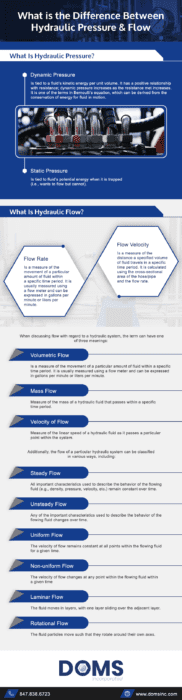Regarding hydraulic systems, two of the key concepts to understand are pressure and flow. While some people use the terms interchangeably, they actually refer to different aspects of hydraulic system operation/performance. Hydraulic flow refers to the movement of the hydraulic fluid through the system. Hydraulic pressure refers to the force of that flow when it encounters resistance. Knowing the difference between them is essential since the approach to flow control differs depending on which factor is targeted.

## What Is Hydraulic Pressure?As indicated above, hydraulic pressure is generated when the flow of hydraulic fluid through the system encounters resistance. However, it is also created by the potential energy of an object on which gravity is acting. Hydraulic pressure produced in the former situation is known as dynamic pressure, while hydraulic pressure produced in the latter situation is known as static pressure.

• Dynamic pressure is tied to a fluid’s kinetic energy per unit volume. It has a positive relationship with resistance; dynamic pressure increases as the resistance met increases. It is one of the terms in Bernoulli’s equation, which can be derived from the conservation of energy for fluid in motion.
• Static pressure is tied to fluid’s potential energy when it is trapped (i.e., wants to flow but cannot).

## What Is Hydraulic Flow?Hydraulic flow is the movement of hydraulic fluid within the system. Two related factors are flow rate and flow velocity

• Flow rate is a measure of the movement of a particular amount of fluid within a specific time period. It is usually measured using a flow meter and can be expressed in gallons per minute or liters per minute.
• Flow velocity is a measure of the distance a specified volume of fluid travels in a specific time period. It is calculated using the cross-sectional area of the hose/pipe and the flow rate.

When discussing flow with regard to a hydraulic system, the term can have one of three meanings:

• Volumetric flow: measure of the volume of a hydraulic fluid that passes
within a specific time period
• Mass flow: measure of the mass of a hydraulic fluid that passes within a specific time period
• Velocity of flow: measure of the linear speed of a hydraulic fluid as it passes a particular point within the system

Additionally, the flow of a particular hydraulic system can be classified in various ways, including:

• Steady flow: all important characteristics used to describe the behavior of the flowing fluid (e.g., density, pressure, velocity, etc.) remain constant over time
• Unsteady flow: any of the important characteristics used to describe the behavior of the flowing fluid changes over time
• Uniform flow: the velocity of flow remains constant at all points within the flowing fluid for a given time
• Non-uniform flow: the velocity of flow changes at any point within the flowing fluid within a given time
• Laminar flow: the fluid moves in layers, with one layer sliding over the adjacent layer
• Rotational flow: the fluid particles move such that they rotate around their own axes